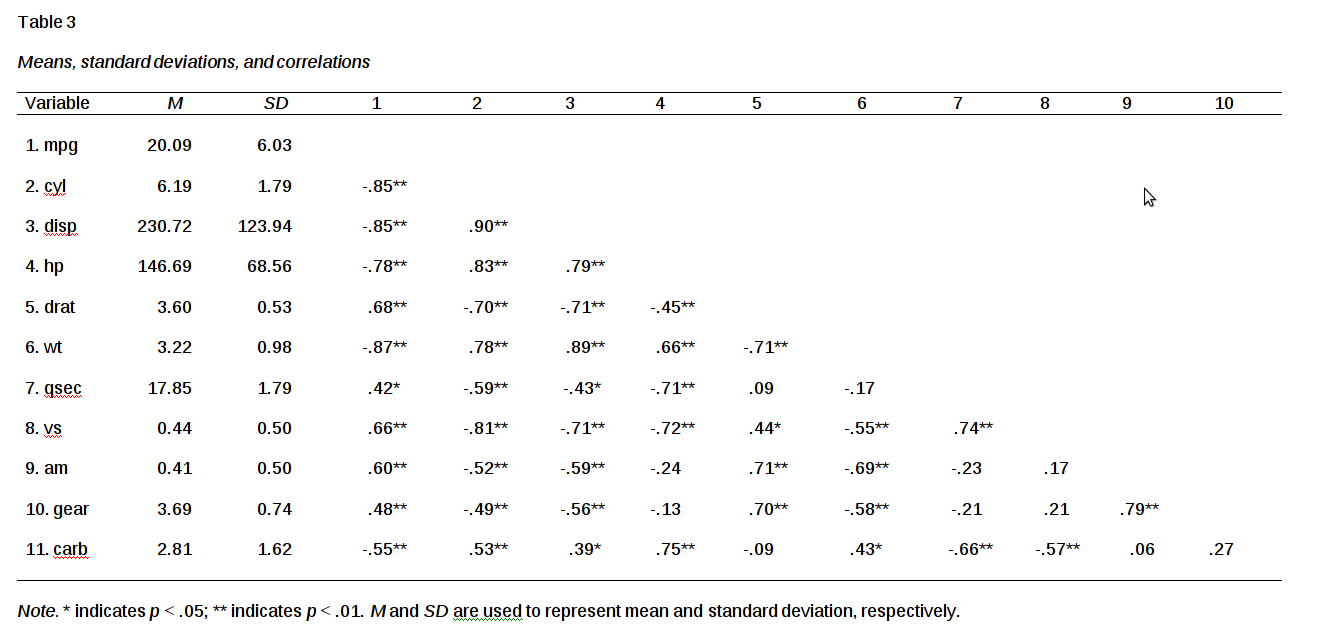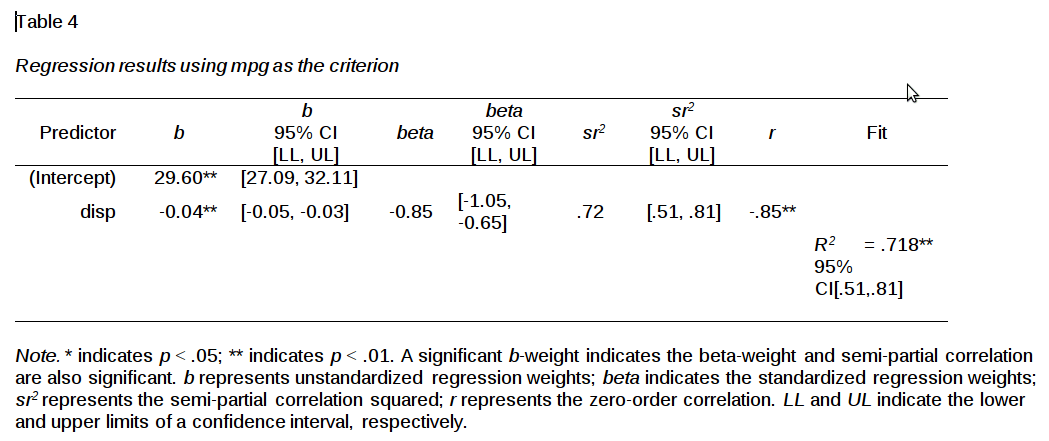# APA Tables in R

Anybody who has ever had to do any writing for academic purposes or in industry has had to deal with APA formatting. The rules and expectations seem to be endless and always changing. If you are able to maneuver the endless list of rules you still have to determine what to report and how when writing an article.

There is a package in R that can at least take away the mystery of how to report ANOVA, correlation, and regression tables. This package is called “apaTables”. In this post, we will look at how to use this package for making tables that are formatted according to APA.

We are going to create examples of ANOVA, correlation, and regression tables using the ‘mtcars’ dataset. Below is the initial code that we need to begin.

``````library(apaTables)
data("mtcars")``````

ANOVA

We will begin with the results of ANOVA. In order for this to be successful, you have to use the “lm” function to create the model. If you are familiar with ANOVA and regression this should not be surprising as they both find the same answer using different approaches. After the “lm” function you must use the “filename” argument and give the output a name in quotations. This file will be saved in your R working directory. You can also provide other information such as the table number and confidence level if you desire.

There will be two outputs in our code. The output to the console is in R. A second output will be in a word doc. Below is the code.

``apa.aov.table(lm(mpg~cyl,mtcars),filename = "Example1.doc",table.number = 1)``
``````##
##
## Table 1
##
## ANOVA results using mpg as the dependent variable
##
##
##    Predictor      SS df      MS      F    p partial_eta2
##  (Intercept) 3429.84  1 3429.84 333.71 .000
##          cyl  817.71  1  817.71  79.56 .000          .73
##        Error  308.33 30   10.28
##  CI_90_partial_eta2
##
##          [.56, .80]
##
##
## Note: Values in square brackets indicate the bounds of the 90% confidence interval for partial eta-squared``````

Here is the word doc outputPerhaps you are beginning to see the beauty of using this package and its functions. The “apa.aov.table”” function provides a nice table that requires no formatting by the researcher.

You can even make a table of the means and standard deviations of ANOVA. This is similar to what you would get if you used the “aggregate” function. Below is the code.

``apa.1way.table(cyl, mpg,mtcars,filename = "Example2.doc",table.number = 2)``
``````##
##
## Table 2
##
## Descriptive statistics for mpg as a function of cyl.
##
##  cyl     M   SD
##    4 26.66 4.51
##    6 19.74 1.45
##    8 15.10 2.56
##
## Note. M and SD represent mean and standard deviation, respectively.
## ``````

Here is what it looks like in wordCorrelation

We will now look at an example of a correlation table. The function for this is “apa.cor.table”. This function works best with only a few variables. Otherwise, the table becomes bigger than a single sheet of paper. In addition, you probably will want to suppress the confidence intervals to save space. There are other arguments that you can explore on your own. Below is the code

``apa.cor.table(mtcars,filename = "Example3.doc",table.number = 3,show.conf.interval = F)``
``````##
##
## Table 3
##
## Means, standard deviations, and correlations
##
##
##   Variable M      SD     1      2      3      4      5      6      7
##   1. mpg   20.09  6.03
##
##   2. cyl   6.19   1.79   -.85**
##
##   3. disp  230.72 123.94 -.85** .90**
##
##   4. hp    146.69 68.56  -.78** .83**  .79**
##
##   5. drat  3.60   0.53   .68**  -.70** -.71** -.45**
##
##   6. wt    3.22   0.98   -.87** .78**  .89**  .66**  -.71**
##
##   7. qsec  17.85  1.79   .42*   -.59** -.43*  -.71** .09    -.17
##
##   8. vs    0.44   0.50   .66**  -.81** -.71** -.72** .44*   -.55** .74**
##
##   9. am    0.41   0.50   .60**  -.52** -.59** -.24   .71**  -.69** -.23
##
##   10. gear 3.69   0.74   .48**  -.49** -.56** -.13   .70**  -.58** -.21
##
##   11. carb 2.81   1.62   -.55** .53**  .39*   .75**  -.09   .43*   -.66**
##
##   8      9     10
##
##
##
##
##
##
##
##
##
##
##
##
##
##
##
##
##   .17
##
##   .21    .79**
##
##   -.57** .06   .27
##
##
## Note. * indicates p < .05; ** indicates p < .01.
## M and SD are used to represent mean and standard deviation, respectively.
## ``````

Here is the word doc resultsIf you run this code at home and open the word doc in Word you will not see variables 9 and 10 because the table is too big by itself for a single page. I hade to resize it manually. One way to get around this is to delate the M and SD column and place those as rows below the table.

Regression

Our final example will be a regression table. The code is as follows

``apa.reg.table(lm(mpg~disp,mtcars),filename = "Example4",table.number = 4)``
``````##
##
## Table 4
##
## Regression results using mpg as the criterion
##
##
##    Predictor       b       b_95%_CI  beta    beta_95%_CI sr2 sr2_95%_CI
##  (Intercept) 29.60** [27.09, 32.11]
##         disp -0.04** [-0.05, -0.03] -0.85 [-1.05, -0.65] .72 [.51, .81]
##
##
##
##       r             Fit
##
##  -.85**
##             R2 = .718**
##         95% CI[.51,.81]
##
##
## Note. * indicates p < .05; ** indicates p < .01.
## A significant b-weight indicates the beta-weight and semi-partial correlation are also significant.
## b represents unstandardized regression weights; beta indicates the standardized regression weights;
## sr2 represents the semi-partial correlation squared; r represents the zero-order correlation.
## Square brackets are used to enclose the lower and upper limits of a confidence interval.
## ``````

Here are the results in wordYou can also make regression tables that have multiple blocks or models. Below is an example

``apa.reg.table(lm(mpg~disp,mtcars),lm(mpg~disp+hp,mtcars),filename = "Example5",table.number = 5)``
``````##
##
## Table 5
##
## Regression results using mpg as the criterion
##
##
##    Predictor       b       b_95%_CI  beta    beta_95%_CI sr2  sr2_95%_CI
##  (Intercept) 29.60** [27.09, 32.11]
##         disp -0.04** [-0.05, -0.03] -0.85 [-1.05, -0.65] .72  [.51, .81]
##
##
##
##  (Intercept) 30.74** [28.01, 33.46]
##         disp -0.03** [-0.05, -0.02] -0.62 [-0.94, -0.31] .15  [.00, .29]
##           hp   -0.02  [-0.05, 0.00] -0.28  [-0.59, 0.03] .03 [-.03, .09]
##
##
##
##       r             Fit        Difference
##
##  -.85**
##             R2 = .718**
##         95% CI[.51,.81]
##
##
##  -.85**
##  -.78**
##             R2 = .748**    Delta R2 = .03
##         95% CI[.54,.83] 95% CI[-.03, .09]
##
##
## Note. * indicates p < .05; ** indicates p < .01.
## A significant b-weight indicates the beta-weight and semi-partial correlation are also significant.
## b represents unstandardized regression weights; beta indicates the standardized regression weights;
## sr2 represents the semi-partial correlation squared; r represents the zero-order correlation.
## Square brackets are used to enclose the lower and upper limits of a confidence interval.
## ``````

Here is the word doc versionConculsion

This is a real time saver for those of us who need to write and share statistical information.

## 3 thoughts on “APA Tables in R”

1.Magno Araújo

That was very helpful. Thanks for sharing. I was unaware of this feature.

2.Romina

“There will be two outputs in our code. The output to the console is in R. A second output will be in a word doc.” Where is the second word doc Output? I don’t find it 🙁
Also the suppressing of confidence intervals doesn’t seem to work anymore, the show.conf.interval=F command gets the notifcation “The ability to suppress reporting of confidene intervals has been deprecated in this version.”

Would be really happy if you could help me. Thank you!

1.Dr. Darrin

You have to open the word doc it should be in your working directory